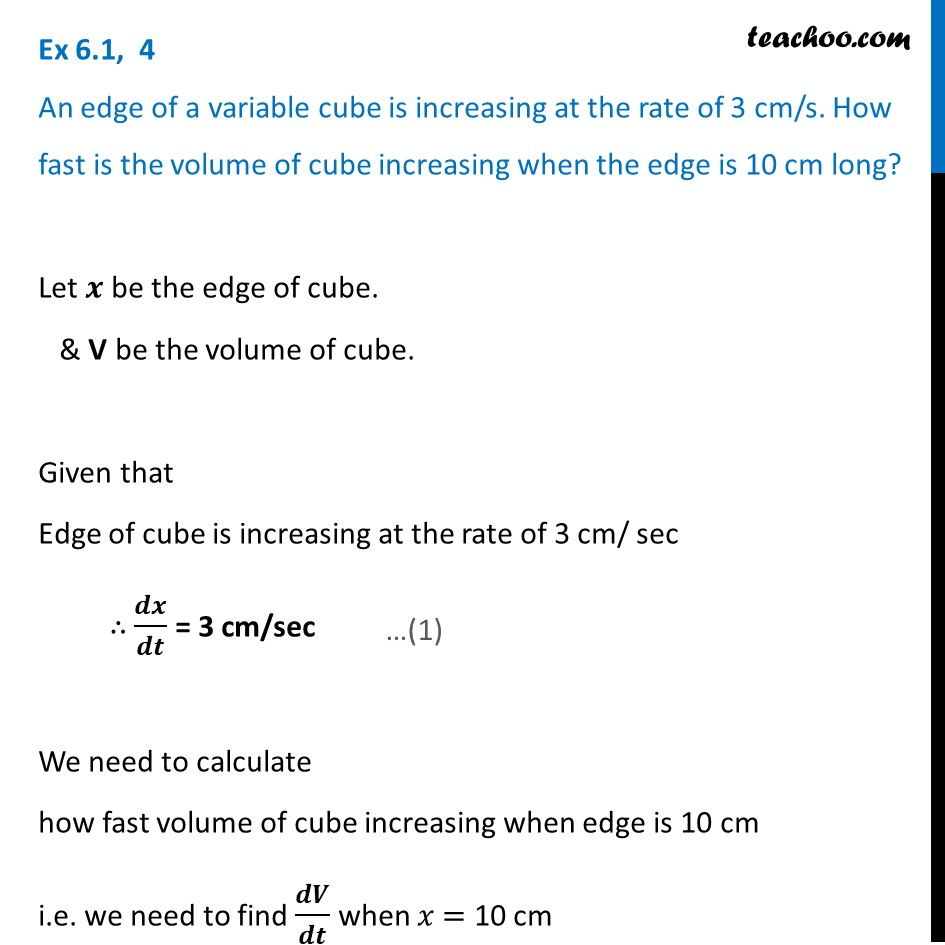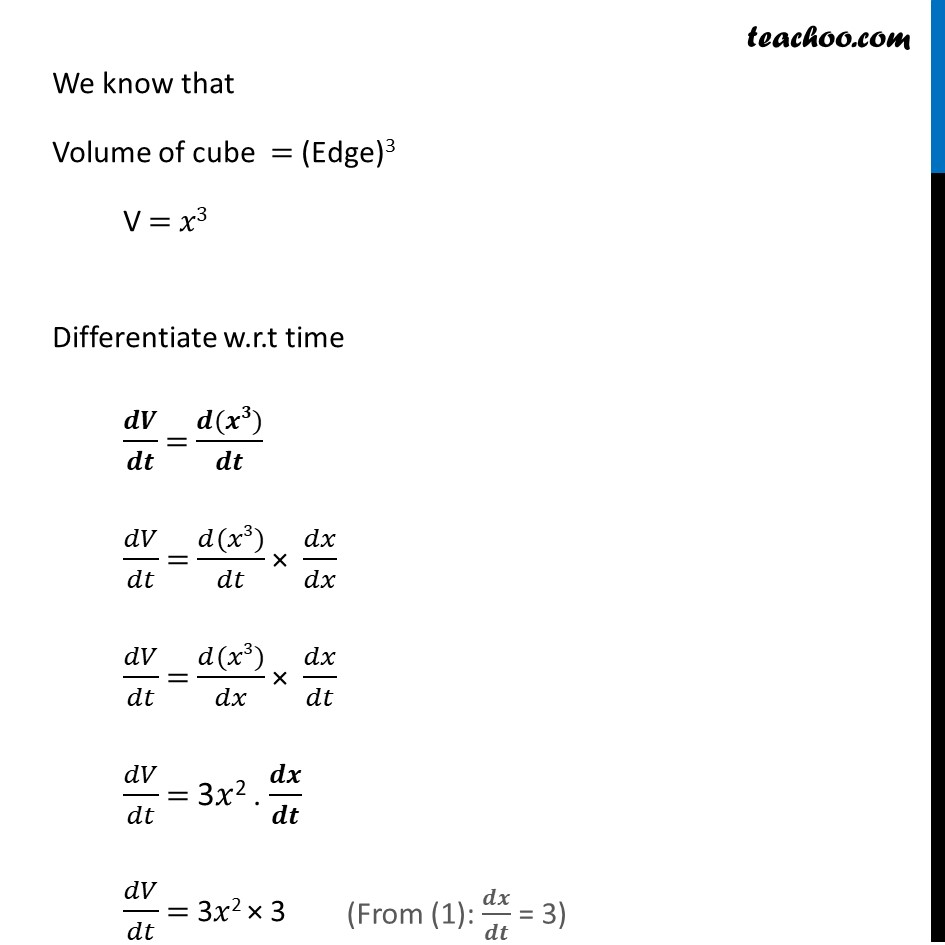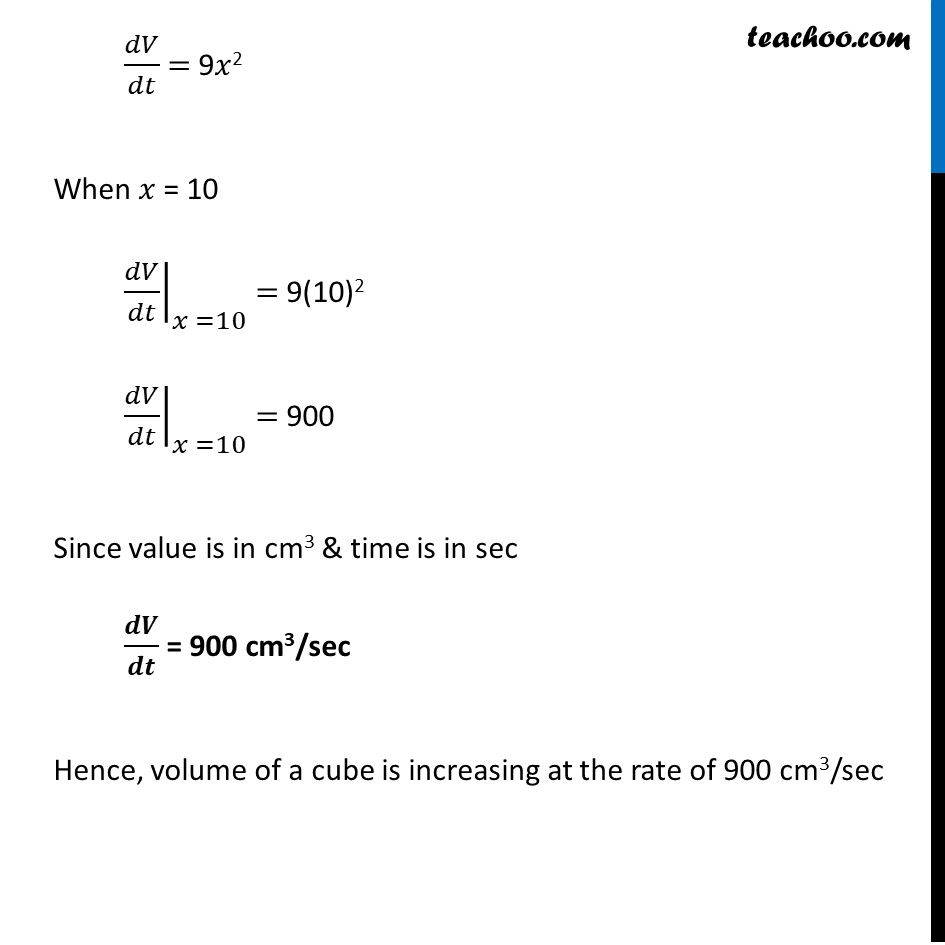Ex 6.1

Chapter 6 Class 12 Application of Derivatives
Serial order wiseLearn in your speed, with individual attention - Teachoo Maths 1-on-1 Class

### Transcript

Ex 6.1, 4 An edge of a variable cube is increasing at the rate of 3 cm/s. How fast is the volume of cube increasing when the edge is 10 cm long?Let 𝒙 be the edge of cube. & V be the volume of cube. Given that Edge of cube is increasing at the rate of 3 cm/ sec ∴ 𝒅𝒙/𝒅𝒕 = 3 cm/sec We need to calculate how fast volume of cube increasing when edge is 10 cm i.e. we need to find 𝒅𝑽/𝒅𝒕 when 𝑥 = 10 cm We know that Volume of cube = (Edge)3 V = 𝑥3 Differentiate w.r.t time 𝒅𝑽/𝒅𝒕 = (𝒅(𝒙𝟑))/𝒅𝒕 𝑑𝑉/𝑑𝑡 = (𝑑(𝑥3))/𝑑𝑡 × 𝑑𝑥/𝑑𝑥 𝑑𝑉/𝑑𝑡 = (𝑑(𝑥3))/𝑑𝑥 × 𝑑𝑥/𝑑𝑡 𝑑𝑉/𝑑𝑡 = 3𝑥2 . 𝒅𝒙/𝒅𝒕 𝑑𝑉/𝑑𝑡 = 3𝑥2 × 3 𝑑𝑉/𝑑𝑡 = 9𝑥2 When 𝑥 = 10 ├ 𝑑𝑉/𝑑𝑡┤|_(𝑥 =10) = 9(10)2 ├ 𝑑𝑉/𝑑𝑡┤|_(𝑥 =10) = 900 Since value is in cm3 & time is in sec 𝒅𝑽/𝒅𝒕 = 900 cm3/sec Hence, volume of a cube is increasing at the rate of 900 cm3/sec